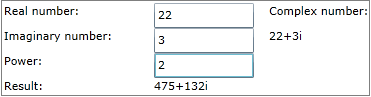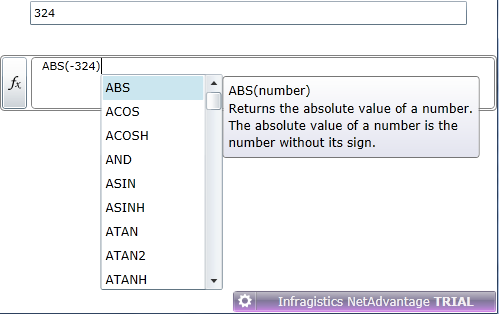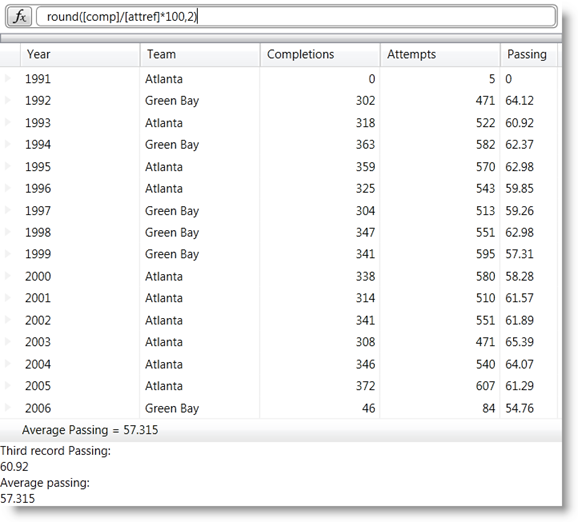# Introducing the XamCalculationManager

Damyan Petev / Monday, October 31, 2011

The XamCalculationManager is one of the controls added to the NetAdvantage XAML controls family with the 11.2 Release. Following our Unified XAML Product Strategy it is a XAML cross-platform tool for creating complex formulas. It also comes with many built-in functions similar to the well-known Microsoft® Excel® functions as well as the option to register you own functions.

The XamCalculationManager is a non-visual component that allows other objects to be sources or result targets for the calculations, including native WPF or Silverlight controls and Infragistics XAML controls. All happens with setting the formula property, which will use the mentioned sources and targets and familiar and custom functions to perform complex calculations. Excel-Style functions supported by the control include common aggregate ones like Sum and Average and also lists with Date and Time, Engineering, Financial, Math, Statistical functions and more. With over 100 built-in functions to help provide powerful functionality it will surely deliver a familiar and enjoyable experience for end users.

There is also an ItemCalculation component which can be used to perform operations on the data bound to it and will expose a collection with properties for the items that can be sources or targets of formulas. It will do the calculations and also expose the results.

Of course there’s a ListCalculator component that can perform calculation on series of data and it can use both Item Calculations on a single item in the list or List Calculations that are executed across all items in the list.

The XamCalculationManager also supports such distinctive features like control over the calculation frequency with asynchronous option to improve performance with large amounts of data and interaction with the also new Formula Editor and integration with the XamDataGrid.

## Getting Started

This might look like almost too much work for a simple calculation but keep in mind that all really depends on the Formula property you set and the rest of the steps would generally remain the same for much more complicated calculations you may have in mind.

After adding the shared Infragistics Silverlight assembly along with the XamCalculationManager one you should also add the Infragistics namespace:

1. xmlns:ig="http://schemas.infragistics.com/xaml"

As mentioned above the control has no visual representation so for example it can be referenced in the Resources depending on the scope required:

1. <UserControl.Resources>
2.     <ig:XamCalculationManager x:Key="CalcManager"/>
3. </UserControl.Resources>

Lets say the goal is to calculate the power of a complex number so there need to be two controls(e.g. textbox) for real and imaginary numbers and one for the power:

1. <TextBox x:Name="real" ig:XamCalculationManager.CalculationManager="{StaticResource CalcManager}"/>
2. <TextBox x:Name="imaginary" ig:XamCalculationManager.CalculationManager="{StaticResource CalcManager}"/>
3. <TextBox ig:XamCalculationManager.CalculationManager="{StaticResource CalcManager}">
4.     <ig:XamCalculationManager.ControlSettings>
5.         <ig:ControlCalculationSettings ReferenceId="power"/>
6.     </ig:XamCalculationManager.ControlSettings>
7. </TextBox>

Should you choose to add it somewhere else the Name property can be used for binding:

1. <!--Adding a XamCalculationManager-->
2. <ig:XamCalculationManager Name="CalcManager"></ig:XamCalculationManager>
3. <!--Registering a TextBox for calculation-->
4. <TextBox x:Name="real" ig:XamCalculationManager.CalculationManager="{Binding ElementName=CalcManager}"/>

At this point all left is a control to display the result:

1. <TextBlock x:Name="Result" ig:XamCalculationManager.CalculationManager="{StaticResource CalcManager}">
2.     <ig:XamCalculationManager.ControlSettings>
3.         <ig:ControlCalculationSettings
4.             Property="Text"
5.             ReferenceId="powResult"
6.             Formula="IMPOWER(COMPLEX([real],[imaginary]),[power])" />
7.     </ig:XamCalculationManager.ControlSettings>
8. </TextBlock>

And the results would look something like this:As demonstrated by the code above formulas can calculate based on the x:Name property of the control. By default the XamCalculationManager uses the x:Name as ReferenceId, but if x:Name is not defined the ReferenceId must be set (see the third Textbox).

### Custom Functions

In case there’s a formula that can’t be constructed with the built-in functions there’s also the option to register a custom one – the CustomCalculationFunction class allows to create new functions by providing their logic:

1. using Infragistics.Calculations;
1. XamCalculationManager calcManager = (XamCalculationManager)this.Resources["CalcManager"];
2. CustomCalculationFunction cplxMagnitude = new CustomCalculationFunction("cplxMagnitude",
3.     (x, y) => (
4.         Complex.Abs(new Complex(x, y))
5.     ));
6. calcManager.RegisterUserDefinedFunction(cplxMagnitude);

Now the ‘cplxMagniude’  can be used in formulas just  like any other function.

1. <TextBox x:Name="customResult" ig:XamCalculationManager.CalculationManager="{StaticResource CalcManager}">
2.     <ig:XamCalculationManager.ControlSettings>
3.         <ig:ControlCalculationSettings
4.             Property="Text"
5.             ReferenceId="customResult"
6.             Formula="cplxMagnitude([n1],[n2])" />
7.     </ig:XamCalculationManager.ControlSettings>
8. </TextBox>

### Item and List Calculators

With those components you can perform calculations on bound data using the XamCalculationManager. The ItemCalculator exposes a collection of ItemCalculations with properties of the specified item that can be source/target of a formula. It will perform the calculations and also expose the results. In addition you can set ReferenceId and use it separately to do some additional computing:

1. <!--Item Calculation-->
2. <ig:ItemCalculatorElement x:Name="itemCalc"
3.                           CalculationManager="{StaticResource CalcManager}"
4.                           Item="{Binding}">
5.     <ig:ItemCalculatorElement.Calculator>
6.         <ig:ItemCalculator ReferenceId="PersonDetailCalc">
7.             <ig:ItemCalculator.Calculations>
8.                 <ig:ItemCalculation ReferenceId="Savings"
9.                                     Formula="([Salary] - [Expenses])"/>
10.                 <ig:ItemCalculation ReferenceId="RentValue"
11.                                     Formula="([Rent] / [Expenses])"/>
12.             </ig:ItemCalculator.Calculations>
13.         </ig:ItemCalculator>
14.     </ig:ItemCalculatorElement.Calculator>
15. </ig:ItemCalculatorElement>
16. <!--Displaying results using the Results dictionary-->
17. <TextBlock x:Name="ResultFromDict" Text="{Binding ElementName=itemCalc, Path=Calculator.Results[Savings].Value}"/>
18. <!--Displaying results using referenceID with the XamCalculationManager-->
19. <TextBlock x:Name="ResultFromRefId"
20.            ig:XamCalculationManager.CalculationManager="{StaticResource CalcManager}">
21.     <ig:XamCalculationManager.ControlSettings>
22.         <ig:ControlCalculationSettings Formula="[PersonDetailCalc/RentValue]"/>
23.     </ig:XamCalculationManager.ControlSettings>
24. </TextBlock>

To perform operations over series of data The ListCalculator would be set up in very similar manner except the fact that it would be bound to an ItemSource rather than a single item and would contain either a collection of ItemCalctulations to be applied separately to each item in the list or ListCalculations that will be performed for all items. For example let’s assume the above code under ‘Item Calculation’ is instead with ListCalculator and what you can now add ListCalculations:

1. <!--This goes inside <ig:ListCalculator> tag-->
2. <ig:ListCalculator.ListCalculations>
3.     <ig:ListCalculation ReferenceId="TotalSavings" Formula="Sum([Savings])"/>
4. </ig:ListCalculator.ListCalculations>

### Named References

With the XamCalculationManager you can also take advantage of  a collection of Named References that can be used to globally store globally defined constants or even results. Each NamedReference should be given a unique reference ID and either a static value or a formula to calculate:

1. <ig:XamCalculationManager x:Key="CalcManager">
2.     <ig:XamCalculationManager.NamedReferences>
3.         <ig:NamedReferenceCollection>
4.             <ig:NamedReference ReferenceId="one" Value="1" />
5.             <ig:NamedReference ReferenceId="half" Value=".5"/>
6.             <ig:NamedReference ReferenceId="twoAndHalf" Formula="2 * [one] + [half]"/>
7.         </ig:NamedReferenceCollection>
8.     </ig:XamCalculationManager.NamedReferences>
9. </ig:XamCalculationManager>

The reference IDs can now be used in different formulas but can also be targets for results to store and can also be accessed in code behind.

### Integration

Besides enabling native WPF and Silverlight as well as Infragistics controls for use with formulas the XamCalculationManager can be used in conjunction with the xamFormulaEditor for truly amazing formula creation experience and in 11.2 (still CTP though) integration with xamDataGrid!A simple textbox registered with the XamCalculationManager and a XamFormulaEditor with Target bound to that textbox.XamDataGrid with Passing column values calculated by XamCalculationManager and Formula Editor.

### So You get…

The cross-platform XamCalculationManager delivers powerful computing capabilities, a familiar experience with many Excel-Style functions along with options to customize by adding you own. It manages all formulas, getting values from sources and setting results to targets. Combined with the XamFormulaEditor to create a great way to let end-users enter their own formulas and even better when implemented with a XamDataGrid to complete the look and feel of an application.

A demo project is available here. Keep in mind asseblies from the 11.2 Release (can be trial) are required.

Also make sure to check out my previous post that has also been updated with a demo project and keep an eye out for more on the new release.